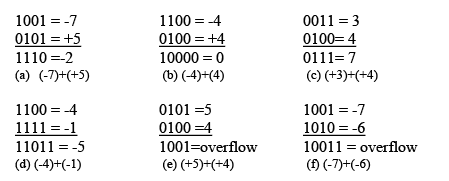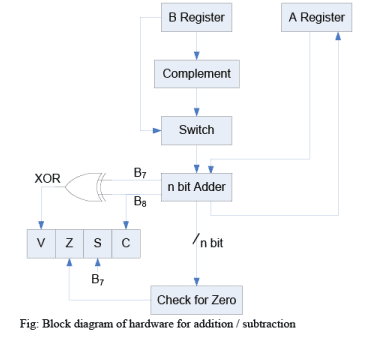Courses

# Addition Algorithm & Subtraction Algorithm - Computer Organization and Architecture | EduRev Notes

## Computer Science Engineering (CSE) : Addition Algorithm & Subtraction Algorithm - Computer Organization and Architecture | EduRev Notes

The document Addition Algorithm & Subtraction Algorithm - Computer Organization and Architecture | EduRev Notes is a part of the Computer Science Engineering (CSE) Course Computer Architecture and Organisation (CAO) - Notes & all.
All you need of Computer Science Engineering (CSE) at this link: Computer Science Engineering (CSE)The first four examples illustrate successful operation if the result of the operation is +ve then we get +ve number in ordinary binary notation. If the result of the operation is –ve we get negative number in twos complement form. Note that in some instants there is carry bit beyond the end of what which is ignored. On any addition the result may larger then can be held in word size being use. This condition is called over flow. When overflow occur ALU must signal this fact so that no attempt is made to use the result. To detect overflow the following rule observed. If two numbers are added, and they are both +ve or both –ve; then overflow occurs if and only if the result has the opposite sign.

The data path and hardware elements needed to accomplish addition and subtraction is shown in figure below. The central element is binary adder, which is presented two numbers for addition and produces a sum and an overflow indication. The binary adder treats the two numbers as unsigned integers. For addition, the two numbers are presented to the adder from two registers A and B. The result may be stored in one of these registers or in the third. The overflow indication is stored in a 1-bit overflow flag V (where 1 = overflow and 0 = no overflow). For subtraction, the subtrahend (B register) is passed through a 2’s complement unit so that its 2’s complement is presented to the adder (a – b = a + (-b)).Offer running on EduRev: Apply code STAYHOME200 to get INR 200 off on our premium plan EduRev Infinity!

,

,

,

,

,

,

,

,

,

,

,

,

,

,

,

,

,

,

,

,

,

;